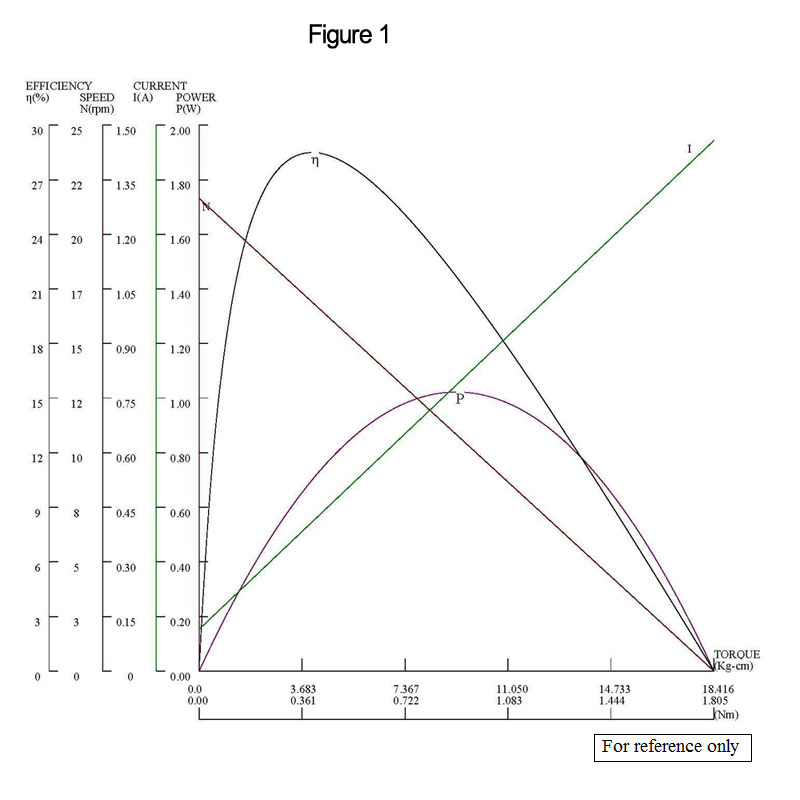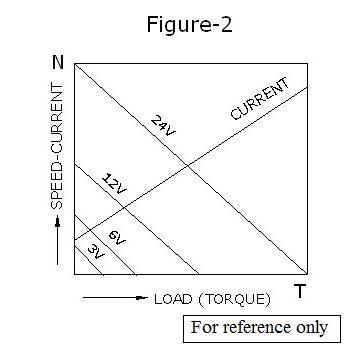# 馬達特性曲線說明

## 瀚港公司簡介

### 馬達特性曲線說明

The relationship between torque (T), speed (N), current (I), efficiency (E), and output power (P), as below figures shown, represents the characteristics of a Hennkwell micro DC gear motor. Figure-1 shows how the loaded torque on the gear motor is in proportion and is directly related to the output speed and current. Figure-2 shows how the no-load speed and starting torque also change proportionally to a different supply voltage. The output speed at a given voltage is parallel to those at other voltage.

As the load on the gear motor increases, speed will decrease accordingly. Additionally, the current (I) is an inverse relationship to torque. The peak of output power (P) and efficiency (E) exist at different torque points, as indicated in figure-1. Output power presents a curve by torque while efficiency (E) decreases straight down beyond the peak normally. Maximum output (Pmax) is at half the starting torque (Ts) point and maximum efficiency exists at a much lower point of torque. A gear motor's basic rating point is lower than its maximum efficiency point. Load torque can be determined by measuring the current drawn when the gear motor is installed in a machine where actual load value is known.

Moreover, the actual operating load torque must be selected lower by a few times than the stall torque. The purpose is to prolong the motor’s life and to bring out the most optimal performance. Besides the full load torque has to operate within the maximum allowable torque limit, although the gear motor could produce an over torque.

The maximum efficiency is generally much lower than the maximum stalled torque, because although the motor can operate at a higher torque than the maximum efficiency torque, it can also shorten the motor’s life due to high current generated. Thus, selecting a motor with a torque that is several times higher than the actual operating torque is strongly recommended.Related Products